Courses

# Design Of Shaft For Variable Load And Based On Stiffness Mechanical Engineering Notes | EduRev

## Mechanical Engineering : Design Of Shaft For Variable Load And Based On Stiffness Mechanical Engineering Notes | EduRev

The document Design Of Shaft For Variable Load And Based On Stiffness Mechanical Engineering Notes | EduRev is a part of the Mechanical Engineering Course Machine Design.
All you need of Mechanical Engineering at this link: Mechanical Engineering

Design of Shaft for variable load

Design of shaft for strength involves certain changes when it is acted upon by variable load. It is required to calculate the mean stress and stress amplitude for all the loads, namely, axial, bending and torsion. Thereafter, any of the design methods for variable load, that is, Soderberg, Goodman or Gerber criteria is utilized. Once again, the familiar design diagram for variable load in terms of the stress amplitude and the mean stress is reproduced below.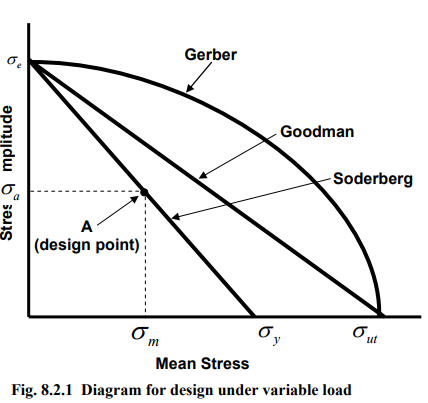A is the design point, for which, the stress amplitude is σa and mean stress is σm. In the Soderberg criterion the mean stress material property is the yield point σy , whereas in the Gerber and the Goodman criteria the material property is the ultimate stress σ ut For the fatigue loading, material property is the endurance limit, σe in reverse bending. The corresponding equations for all the three above criteria are given as,

Goodman criterion: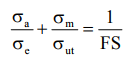Soderberg criterion: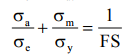Gerber criterion: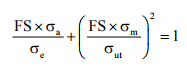(8.2.1)

Where,

σa = Stress amplitude;

σe = Endurance limit;

σm = Mean stress;

σy = Yield point;
σut = Ultimate stress and FS= factor of safety.

Similar equation (8.2.1) also can be written for the shear stress.

For the design of shaft, it is most common to use the Soderberg criterion. Hence, we shall limit our discussion only to Soderberg criterion.

Normal stress equation is given as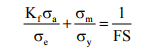multiplying by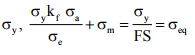(8.2.2)

Similarly, shear stress equation is given as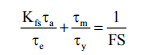multiplying by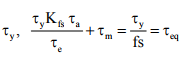(8.2.3)

In equations (8.2.2) and (8.2.3), to consider the effect of variable load, the normal stress amplitude, σa is multiplied by the fatigue stress concentration factor, Kand the corresponding term, shear stress amplitude is multiplied by a fatigue stress concentration factor in shear, Kfs.

The physical significance of equations (8.2.2) and (8.2.3) is that, the effect of variable stress on the machine member (left hand side of the equations), has been effectively defined as an equivalent static stress. Therefore, the problem is treated as a design for static loads. Here, σe or τe are equivalent to allowable stress,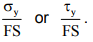Hereafter, conventional failure theories can be used to complete the design.

Maximum shear stress theory

It states that a machine member fails when the maximum shear stress at a point exceeds the maximum allowable shear stress for the shaft material. Therefore,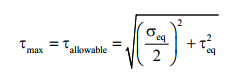(8.2.4)

substitution of σeq and τeq from (8.2.3) will give the required shaft diameter.

Design based on Stiffness

In addition to the strength, design may be based on stiffness. In the context of shaft, design for stiffness means that the lateral deflection of the shaft and/or angle of twist of the shaft should be within some prescribed limit. Therefore, design for stiffness is based on lateral stiffness and torsional rigidity.

Lateral stiffness

Let us consider a beam loaded as shown in Fig.8.2.2. The beam deflects by δ due to the load P. So the requirement for the design is that where, one has to limit the deflection δ. Hence, the design procedure is as follows,

Determine the maximum shaft deflection, using any of the following methods,

Integration method Moment-area method, or Energy method (Theorem of Castigliano)

Now, the deflection, δ = f (applied load, material property, moment of inertia and given dimension of the beam).

From the expression of moment of inertia, and known design parameters, including δ , shaft dimension is obtained.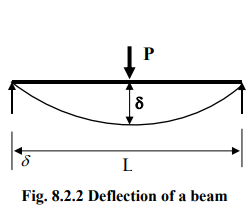Torsional rigidity

To design a shaft based on torsional rigidity, the limit of angle of twist should be known. The angle of twist is given as follows,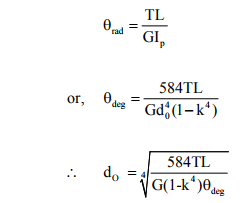(8.2.5)

Where,

θ = angle of twist
L = length of the shaft
G = shear modulus of elasticity
Ip = Polar moment of inertia

The limiting value of θ varies from 0.3 deg/m to 3 deg/m for machine tool shaft to line shaft respectively. With the knowledge of design parameters, the shaft dimension can be obtained from (8.2.5).

A note on critical speed of rotating shaft

Critical speed of a rotating shaft is the speed where it becomes dynamically unstable. It can be shown that the frequency of free vibration of a non-rotating shaft is same as its critical speed.

The equation of fundamental or lowest critical speed of a shaft on two supports is,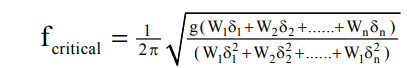(8.2.6)

Where,

W1, W2…. : weights of the rotating bodies
δ1, δ2 …. : deflections of the respective bodies

This particular equation (8.2.6) has been derived using the following assumption.

Assumptions:

The shaft is weightless
The weights are concentrated and
Bearings/supports are not flexible

Where

W1,W2                 :  Weights of the rotating bodies

δ1 and  δ2             : Deflections of the respective bodies

The operating speed of the shaft should be well above or below a critical speed value. There are number of critical speeds depending upon number of rotating bodies.

Sample problem

Design a solid shaft of length 1m, carrying a load of 5 kN at the center and is simply supported as shown in figure. The maximum shaft deflection is 1mm. E=200GPa.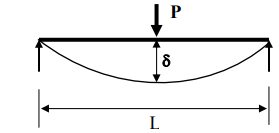Solution

The maximum deflection of the shaft is given as,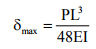where, for a solid shaft,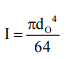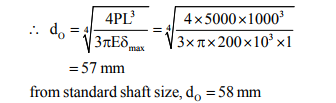This problem is not a complete one. The magnitude of torque on the shaft is not specified. The design calculations should be first based on strength, where, both bending moment and torsion are required. With the given limits of lateral deflection and angular twist, the design should be checked.

Offer running on EduRev: Apply code STAYHOME200 to get INR 200 off on our premium plan EduRev Infinity!

## Machine Design

57 videos|71 docs|102 tests

,

,

,

,

,

,

,

,

,

,

,

,

,

,

,

,

,

,

,

,

,

;